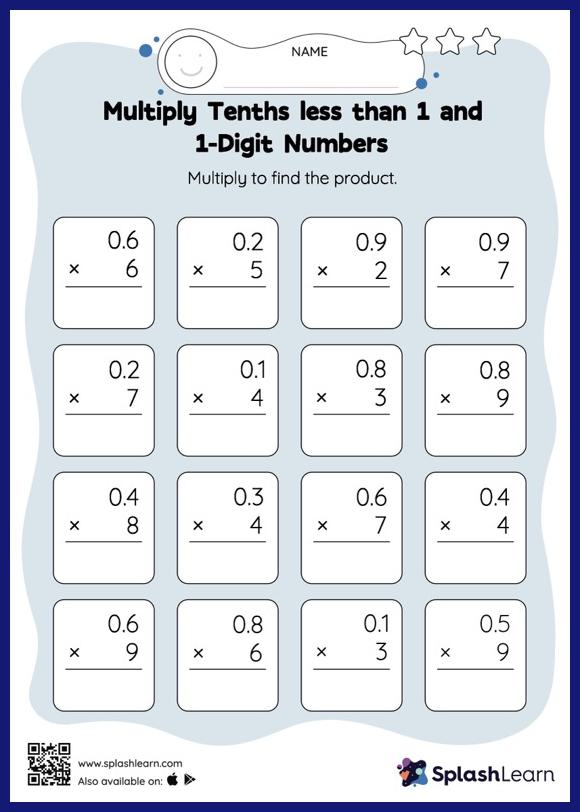# Multiply Tenths less than 1 and 1-Digit Numbers: Vertical Multiplication Worksheet

Home > Multiply Tenths less than 1 and 1-Digit Numbers: Vertical MultiplicationThis worksheet invites students to multiply tenths less than 1 and 1-digit numbers, which helps them become proficient in multiplication. When multiplying a decimal by a one-digit number, students may ignore the decimal point during calculations and bring it back when writing the result. This multiply tenths less than 1 and 1-digit numbers worksheet provides plenty of practice with this concept.